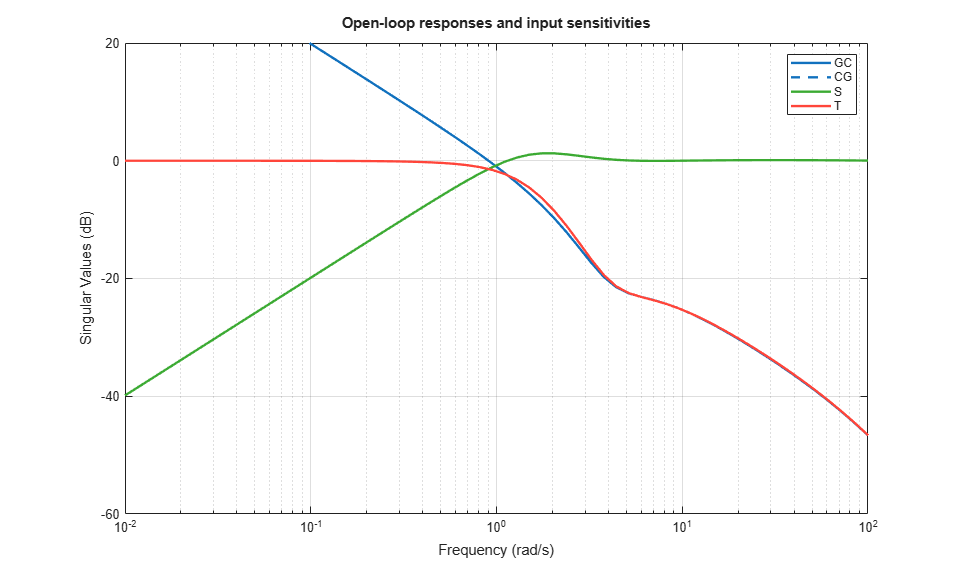# loopview

Graphically analyze results of control system tuning using `slTuner` interface

## Syntax

``loopview(st,controls,measurements)``
``loopview(st,info)``

## Description

example

````loopview(st,controls,measurements)` plots characteristics of the control system described by the `slTuner` interface `st`. Use `loopview` to analyze the performance of a tuned control system you obtain using `looptune`.`loopview` plots: The gains of the open-loop frequency response measured at the plant inputs (`controls` analysis points) and at plant outputs (`measurements` analysis points)The (largest) gain of the sensitivity and complementary sensitivity functions at the plant inputs or outputs```

example

````loopview(st,info)` uses the `info` structure returned by `looptune` and also plots the target and tuned values of tuning constraints imposed on the system. Use this syntax to assist in troubleshooting when tuning fails to meet all requirements.Additional plots with this syntax include: Normalized multi-loop disk margins at the plant inputs and outputs. For more information about disk margins, see Stability Analysis Using Disk Margins (Robust Control Toolbox).Target vs. achieved response for any additional tuning goal you used with `looptune`.```

## Examples

collapse all

Tune the Simulink® model, `rct_engine_speed`, to achieve a specified settling time. Use `loopview` to graphically analyze the tuning results.

Open the model.

```mdl = 'rct_engine_speed'; open_system(mdl); ```Create an `slTuner` interface for the model and specify the PID Controller block to be tuned.

```st0 = slTuner(mdl,'PID Controller'); ```

Specify a requirement to achieve a 2 second settling time for the `Speed` signal when tracking the reference signal.

```req = TuningGoal.Tracking('Ref','Speed',2); ```

Tune the PID Controller block.

```addPoint(st0,'u') control = 'u'; measurement = 'Speed'; wc = 1; [st1,gam,info] = looptune(st0,control,measurement,wc); ```
```Final: Peak gain = 0.979, Iterations = 4 Achieved target gain value TargetGain=1. ```

View the response of the model for the tuned block values.

```loopview(st1,control,measurement); ```Compare the performance of the tuned block against the tuning goals.

```figure loopview(st1,info); ```## Input Arguments

collapse all

Interface for tuning control systems modeled in Simulink, specified as an `slTuner` interface.

Controller output name, specified as one of the following:

• Character vector — Name of an analysis point of `st`.

You can specify the full name or any portion of the name that uniquely identifies the analysis point among the other analysis points of `st`.

For example, `'u'`.

• Cell array of character vectors — Multiple analysis point names.

For example, `{'u','y'}`.

Measurement signal name, specified as one of the following:

• Character vector — Name of an analysis point of `st`.

You can specify the full name or any portion of the name that uniquely identifies the analysis point among the other analysis points of `st`.

For example, `'u'`.

• Cell array of character vector — Multiple analysis point names.

For example, `{'u','y'}`.

Detailed information about each optimization run, specified as the structure returned by `looptune`.

## Alternative Functionality

For analyzing Control System Toolbox™ models tuned with `looptune`, use `loopview`.

## Version History

Introduced in R2014a